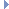﻿ MGeometryIndexMapping Class Reference

# MGeometryIndexMapping Class Reference

MGeometryIndexMapping Class ReferenceRelated help topics:

`#include <MHWGeometry.h>`

## Class Description

A mapping of geometry index.

This class represents the index mapping of the multiple objects concatenated into a single render item.

When multiple objects are compatible, their geometry can be consolidated into a single render item, to provide better performance by concatening their index and vertex buffers.

The Geometry Index Mapping can be used to get the indexing details of the concatenated geometries to be able to render them separately.Examples:

## Public Member Functions

MGeometryIndexMapping ()
Constructor.

~MGeometryIndexMapping ()
Destructor.

int geometryCount () const
Get the number of geometry described by the mapping. More...

MDagPath dagPath (int geometryIdx) const
Get the MDagPath of a geometry. More...

MObject component (int geometryIdx) const
Get the component of a geometry. More...

int indexStart (int geometryIdx) const
Get the index start of a geometry. More...

int indexLength (int geometryIdx) const
Get the index length of a geometry. More...

## Member Function Documentation

 int geometryCount ( ) const

Get the number of geometry described by the mapping.

Returns
The number of geometryExamples:
 MDagPath dagPath ( int geometryIdx ) const

Get the MDagPath of a geometry.

Parameters
 [in] geometryIdx The index the geometry
Returns
The path to the instance of the object's geometryExamples:
 MObject component ( int geometryIdx ) const

Get the component of a geometry.

Parameters
 [in] geometryIdx The index the geometry
Returns
The component of the geometry. This will be null if there is no componentExamples:
 int indexStart ( int geometryIdx ) const

Get the index start of a geometry.

The index start represents the offset of the geometry index data in the index buffer of the consolidated render item

Parameters
 [in] geometryIdx The index the geometry
Returns
The index start of the geometryExamples:
 int indexLength ( int geometryIdx ) const

Get the index length of a geometry.

The index length represents the length of the geometry index data in the index buffer of the consolidated render item

Parameters
 [in] geometryIdx The index the geometry
Returns
The index length of the geometryExamples:

The documentation for this class was generated from the following files:
• MHWGeometry.h
• MHWGeometry.cpp